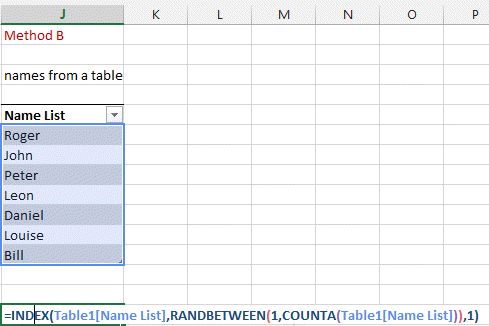Generating random number and Selecting random cell in a table

Excel has a random generator and this one allows you to select randomly in list of names or cell.

Here we show you how this is done.

First lets look at the 2 functions allowing random numbers generation.

RAND(): gives you a number (floating) between 0 and 1. It will have until 15 digits behind the coma. Like 0.123456789012345

RANDBETWEEN(min,max): generates a number between the number min and max. This will be an integer.

Here some examples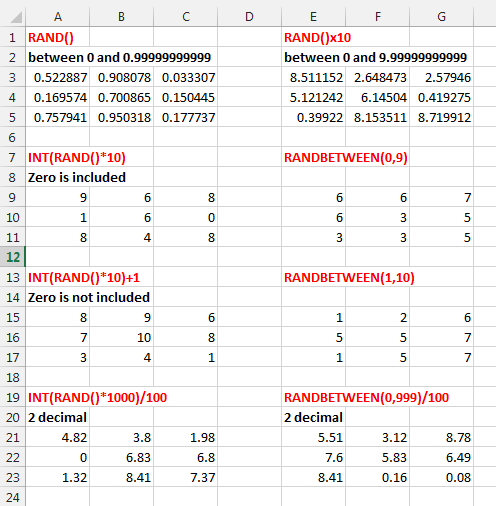You can see an application of the RAND function in the example where we try to calculate the number PI or in the Love Phrase generator  and the psychedelic application.

Lets now select random cells in a list

Two methods at least exist.

METHOD 1 (the complicated one)

Here a list of names and we want to select a random name in this list like in A15 where Roger was selected.

TIP: Press F9 to refresh you list or to generate new numbers.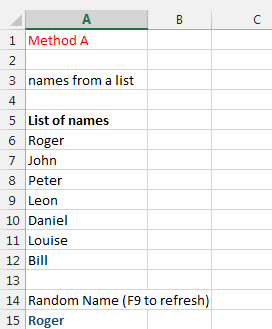First we have to know how many names there is. If you know already and the list never changes just pass this step.

Otherwise, the number of non-empty cells between A6 and A12 can be given by COUNTA(A6:A12)

Then we have to get a random number between the A6 and A12.

So we use RANDBETWEEN(1,COUNTA(A6:A12))

and finally we can choose a name. This can be done using the CHOOSE function

=CHOOSE(rank of the name to choose (1 or 2 or 3, ...) , name1, name2, name3, name4, .......)

This will select a name among the listed names (name1, name2, ...).

The name Rank is chosen randomnly with the randbetween function.

=CHOOSE(      RANDBETWEEN(1,COUNTA(A6:A12)),    A6,A7,A8,A9,A10,A11,A12       )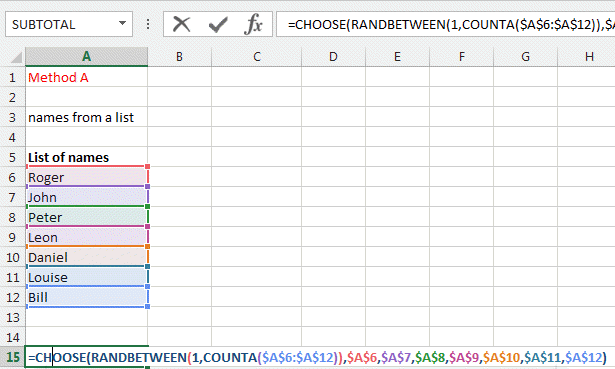METHOD 2 (simple one)

We use the same list but we make a table out of it by using the FORMAT as Table tool in the Home ribbon.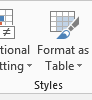Then we can use the INDEX function to select one item in a list of items.

INDEX(Range, row, column)

For example

INDEX(G9:J15,5,3) or

INDEX(Table2,5,3) if you created a table called Table2

Our table is defined and in the index we enter the first column.

As the row index is like in method 1, the RANDBETWEEN function.

INDEX( Table1[Name List] , RANDBETWEEN(1,COUNTA(Table1[Name List])),1)

as there is only 1 column, you can write this like INDEX( Table1 , RANDBETWEEN(1,COUNTA(Table1)),1)

The 1 at the end defines the first column but in our case we would not need it.